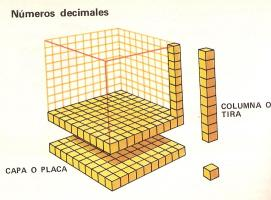1. Decimal numbers1. Introduction.

In the cube there are 10 layers or plates. Each plate is the tenth part of the cube: 1/10 = 0.1.
A cube has 10 layers; 1 unit = 10 tenths.
The cube has 100 columns or strips. Each strip is the hundredth part of the cube: 1/100 = 0.01.
A cube has 100 strips; 1 unit = 100 hundredth.
Each plate has 10 strips: 1 tenth = 10/100.
The cube is composed of 1000 cubes. Each cube is the thousandth part of the cube: 1/1000 = 0.001.

1 unit = 1000 thousandths
1 unit = 10 tenths, 1 tenth = 10 hundredths, 1 hundredth = 10 thousandths.

Write in thousandths these decimals

 1 unit = 1 tenth = 1 hundredth = 1 tenth = 1 unit = 1 hundredth = 1 unit = 1 unit = 1 unit =

2. The tenths

A cube has 10 plates. One unit = 10 tenths. 1 tenth = 0.1.
The decimal numbers have an integer part separated by a comma of the decimal part.
Example: 2.7 it is read: two integers and seven tenths or two comma seven.

What number corresponds for this expression?

 One integer and six tenths = Two integers and five tenths = Seven integers and eight tenths = Zero integers and two tenths = Fourteen integers and three tenths = One hundred and seven integers and nine tenths =

# 3. The hundredths

The cube has 100 columns or strips.
1 unit = 100 hundredths. 1 hundredth = 0.01
1.52 it is read: one integer and fifty-two hundredths or one comma fifty- two.

 One integer and forty-two hundredths = Two integers and twenty two hundredths = Fourteen integers and three hundredths = Thirteen integers and seven tenths = Eight integers and five hundredths = One hundred and seven integers and six tenths =

# 4. The thousandths

The little cube has 1000 cubes. Each little cube is the thousandth part of little cube.
1 unit = 1000 thousandths. 1 thousandth = 0.001
6.125 it is read: Six integers and one hundred twenty-five thousandths or six comma one hundred twenty-five.

 Five integers and two hundred twenty-three thousandths = Seven integers and thirty two hundredths = Fourteen integers and five tenths = One hundred seven integers and eight hundredths = Three thousand integers and three thousandths = Twenty Integers and two hundred twenty seven thousandths= Seventy integers and five tenths = Sixty integers and six hundredths = One hundred and eight integers and four tenths = Six integers and three hundredths =

 | Educational applications | Mathematics | In Spanish |  To print

®Arturo Ramo García.-Record of intellectual property of Teruel (Spain) No 141, of 29-IX-1999
Plaza Playa de Aro, 3, 1ş DO 44002-TERUEL